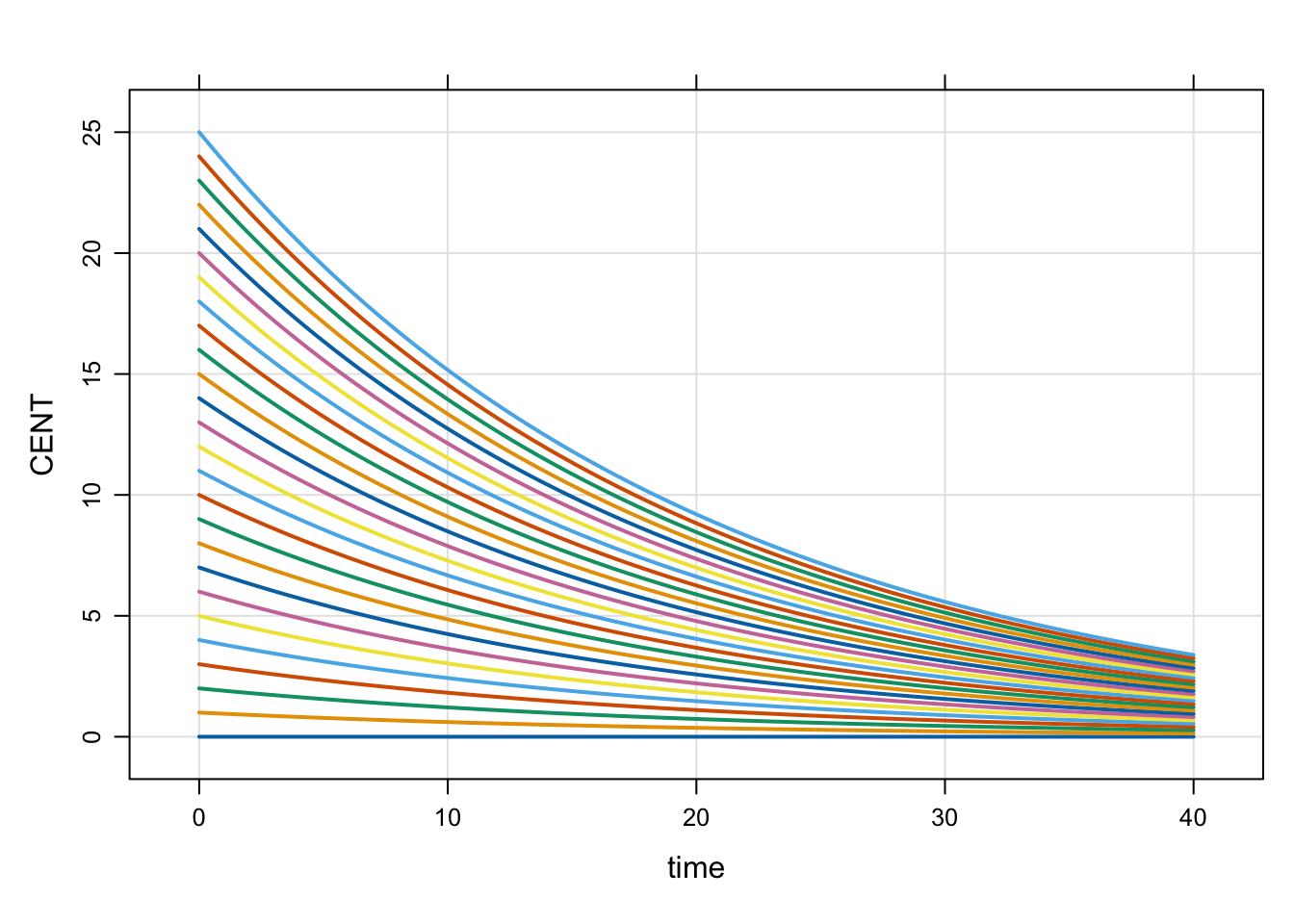# Set initial conditions

There are several ways to set initial conditions in mrgsolve. Find out how this can work to your advantage when planning your simulations.

initial conditions
Author

Kyle Baron

Published

January 1, 2016

There are several ways to set initial conditions in mrgsolve. Find out how this can work to your advantage when planning your simulations.

``````library(mrgsolve)
options(mrgsolve.soloc = "build")``````

There are two commonly-used ways to set initial conditions: in `\$MAIN` and in the initial condition list.

## 1.1 Set initials in `\$MAIN`

For a compartment called `CMT`, there is a variable available to you called `CMT_0` that you can use to set the initial condition of that compartment in `\$MAIN`. For example:

``````code <- '
\$PARAM KIN = 200, KOUT = 50

\$CMT RESP

\$MAIN
RESP_0 = KIN/KOUT;
'``````

This is the most commonly-used way to set initial conditions: the initial condition for the `RESP` compartment is set equal to `KIN` divided by `KOUT`. If you had a parameter called `BASE`, you could also write `RESP_0 = BASE;`. In these examples, we’re using data items from `\$PARAM`. But the initial condition could be set to any numeric value in the model, including individual parameters derived from parameters, covariates, and random effects. Note that you should never declare `RESP_0` (e.g. `double RESP_0`): it just appears for you to use.

## 1.2 Set initials in the `init` list

You can also set initial conditions in the initials list. Most commonly, this means declaring compartments with `\$INIT` rather than `\$CMT`. For example

``````code <- '
\$INIT RESP = 4
'``````

This method gets us the same result as the previous example, however the initial condition now is not a derived value, but it is coded as a number. Alternatively, you could declare a compartment via `\$CMT` and update later (see next).

We can update this value later like this

``mod <- mcode_cache("init_up", code)``
``Loading model from cache.``
``init(mod)``
``````
Model initial conditions (N=1):
name       value . name    value
RESP (1)   4     | . ...   .    ``````
``init(mod, RESP=8) %>% init``
``````
Model initial conditions (N=1):
name       value . name    value
RESP (1)   8     | . ...   .    ``````

This method is commonly used to set initial conditions in large QSP models where the compartment starts out as some known or assumed steady state value.

## 1.3 Don’t use initial conditions as a dosing mechanism

Using an initial condition to put a starting dose in a compartment is not recommended. Always use a dosing event for that.

The following is from a wiki post I did on the topic. It’s pedantic. But hopefully helpful to learn what `mrgsolve` is doing for those who want to know.

• `mrgsolve` keeps a base list of compartments and initial conditions that you can update either from `R` or from inside the model specification
• When you use `\$CMT`, the value in that base list is assumed to be 0 for every compartment
• `mrgsolve` will by default use the values in that base list when starting the problem
• When only the base list is available, every individual will get the same initial condition
• You can override this base list by including code in `\$MAIN` to set the initial condition
• Most often, you do this so that the initial is calculated as a function of a parameter
• For example, `\$MAIN RESP_0 = KIN/KOUT;` when `KIN` and `KOUT` have some value in `\$PARAM`
• This code in `\$MAIN` overwrites the value in the base list for the current `ID`
• For typical PK/PD type models, we most frequently initialize in `\$MAIN`
• This is equivalent to what you might do in your NONMEM model
• For larger systems models, we often just set the initial value via the base list

## 2.1 Make a model only to examine `init` behavior

Note: `IFLAG` is my invention only for this demo. The demo is always responsible for setting and interpreting the value (it is not reserved in any way and `mrgsolve` does not control the value).

For this demo

• Compartment `A` initial condition defaults to 0
• Compartment `A` initial condition will get set to `BASE` only if `IFLAG > 0`
• Compartment `A` always stays at the initial condition (the system doesn’t advance)
``````code <- '
\$PARAM BASE=200, IFLAG = 0

\$CMT A

\$MAIN
if(IFLAG > 0) A_0 = BASE;

\$ODE dxdt_A = 0;
'``````
``mod <- mcode_cache("init_long",code)``
``Loading model from cache.``
``dplot <- function(x) plot(x,ylim=c(0,400))``

Check the initial condition

``init(mod)``
``````
Model initial conditions (N=1):
name    value . name    value
A (1)   0     | . ...   .    ``````

Note:

• We used `\$CMT` in the model spec; that implies that the base initial condition for `A` is set to 0
• In this chunk, the code in `\$MAIN` doesn’t get run because `IFLAG` is 0
• So, if we don’t update something in `\$MAIN` the initial condition is as we set it in the base list
``mod %>% mrgsim %>% dplot``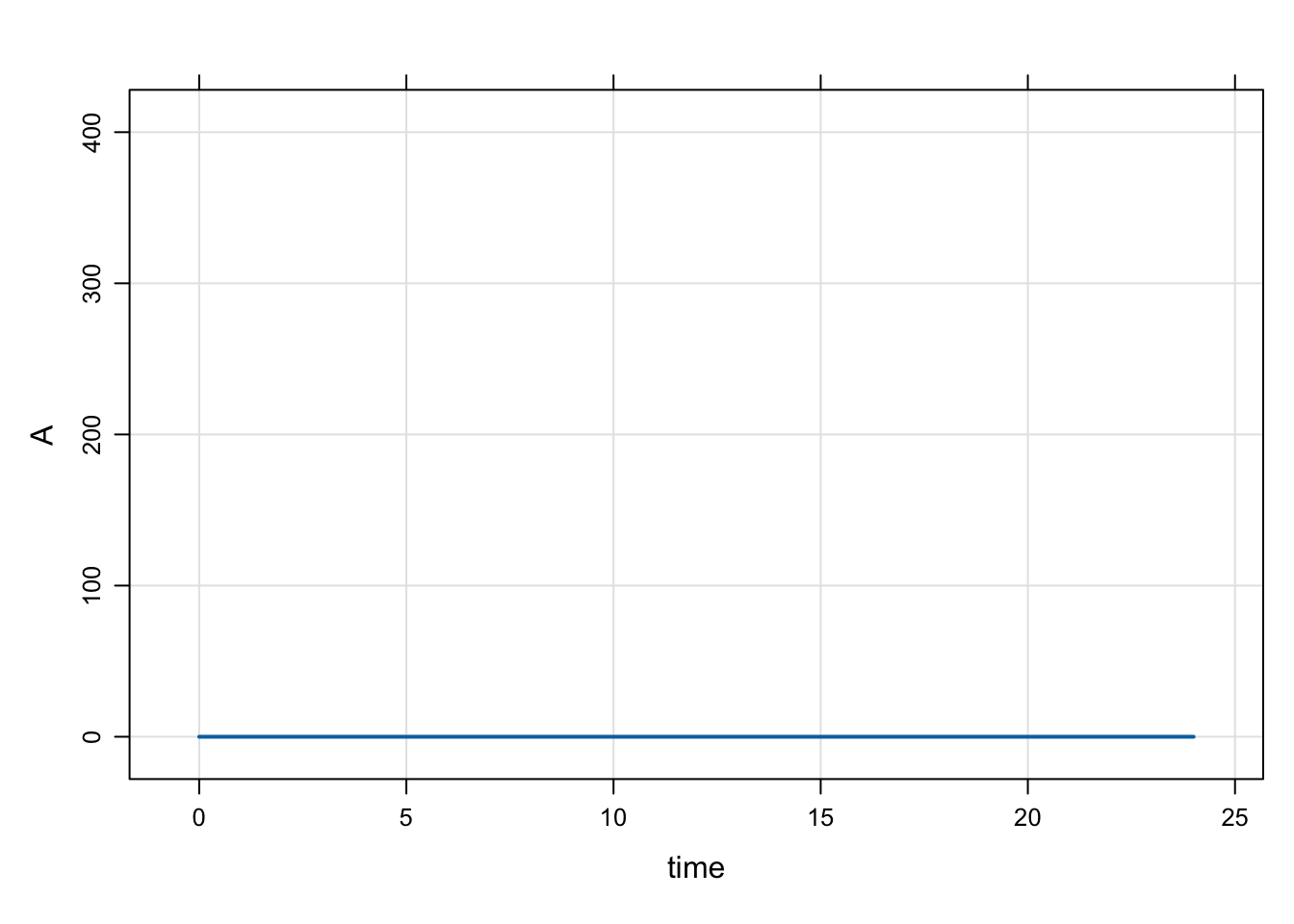Next, we update the base initial condition for `A` to 100

Note:

• The code in `\$MAIN` still doesn’t get run because `IFLAG` is 0
``mod %>% init(A = 100) %>% mrgsim %>% dplot``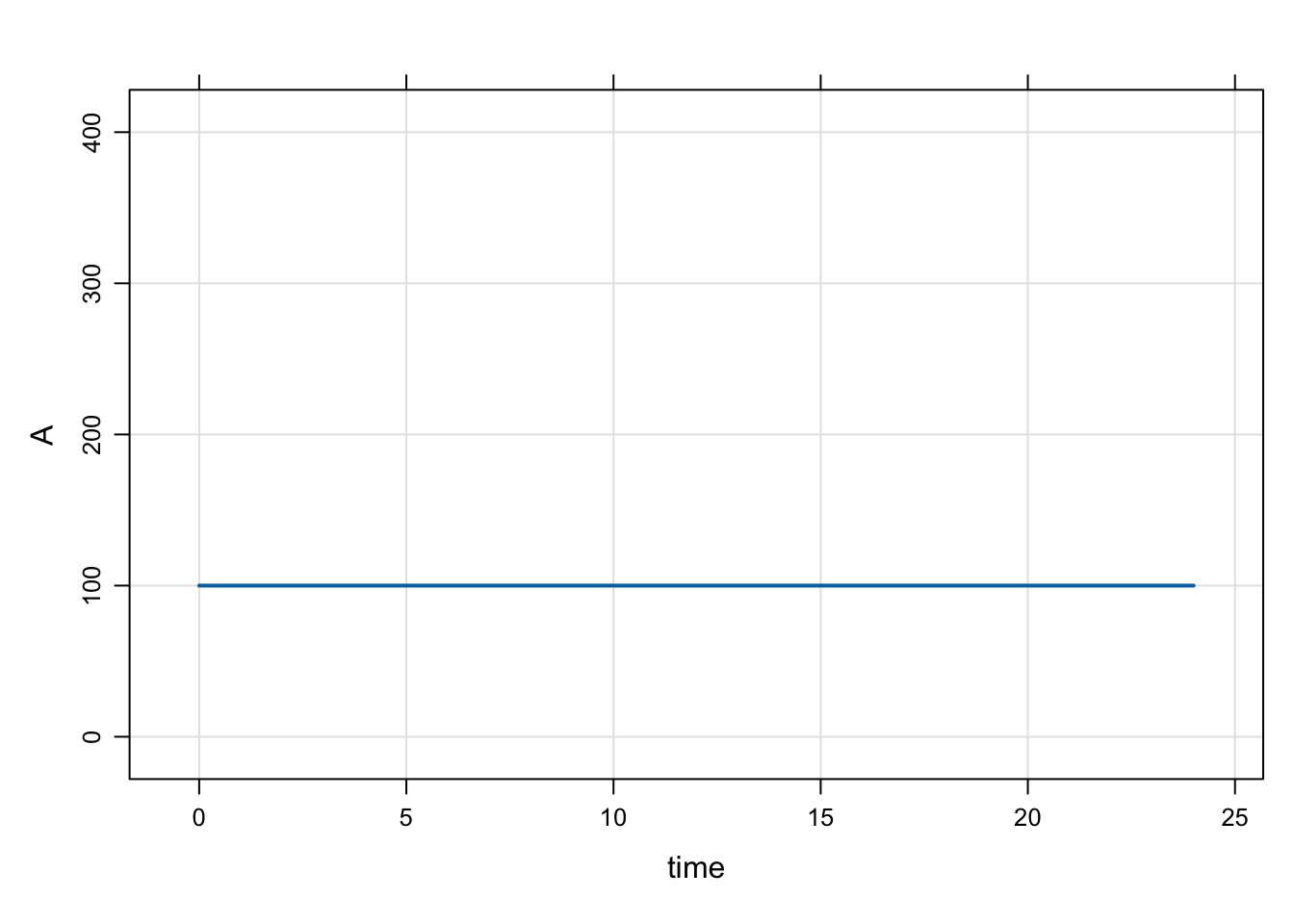Now, turn on `IFLAG`

Note:

• Now, that code in `\$MAIN` gets run
• `A_0` is set to the value of `BASE`
``mod %>% param(IFLAG=1) %>% mrgsim %>% dplot``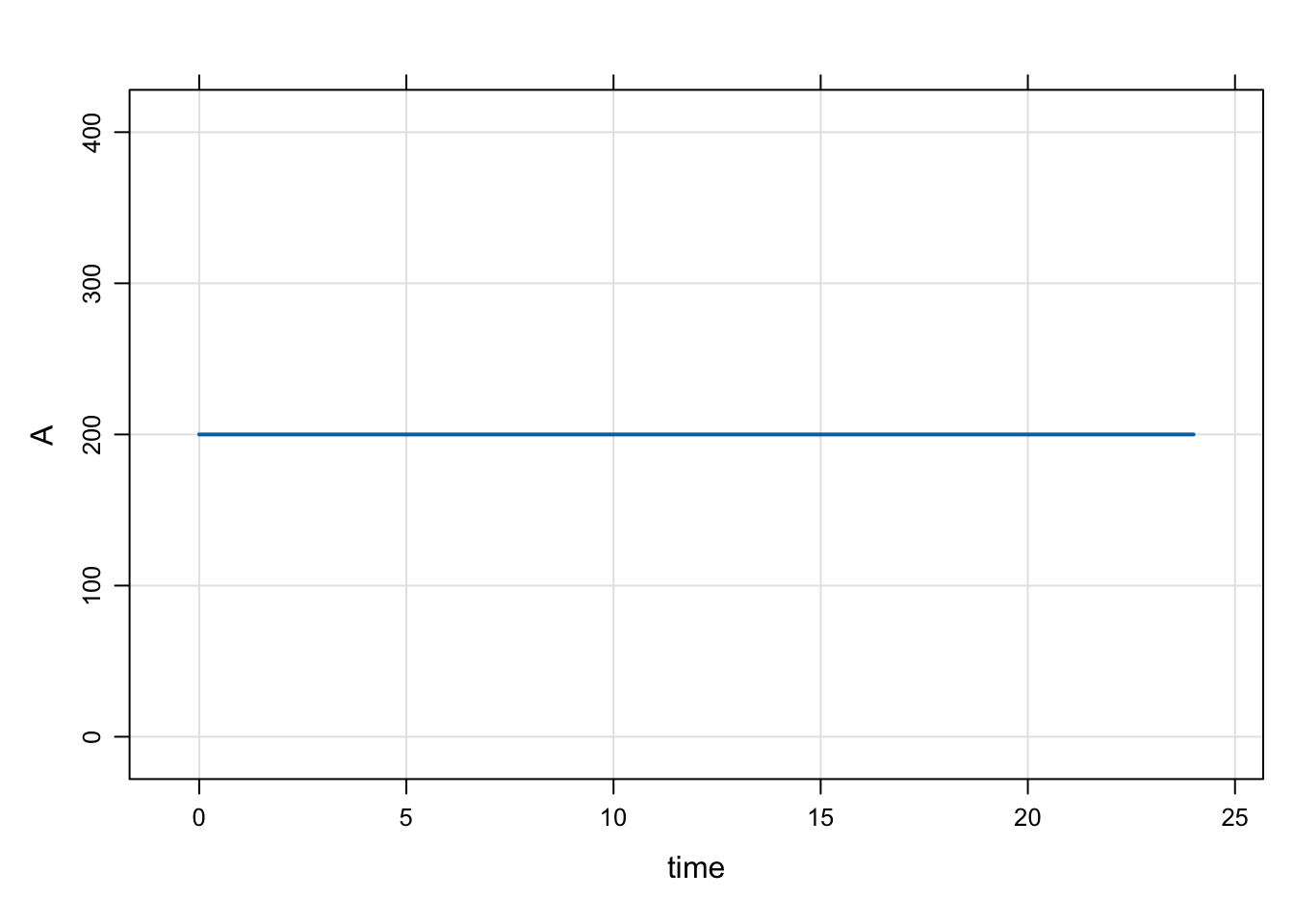``mod %>% param(IFLAG=1, BASE=300) %>% mrgsim %>% dplot``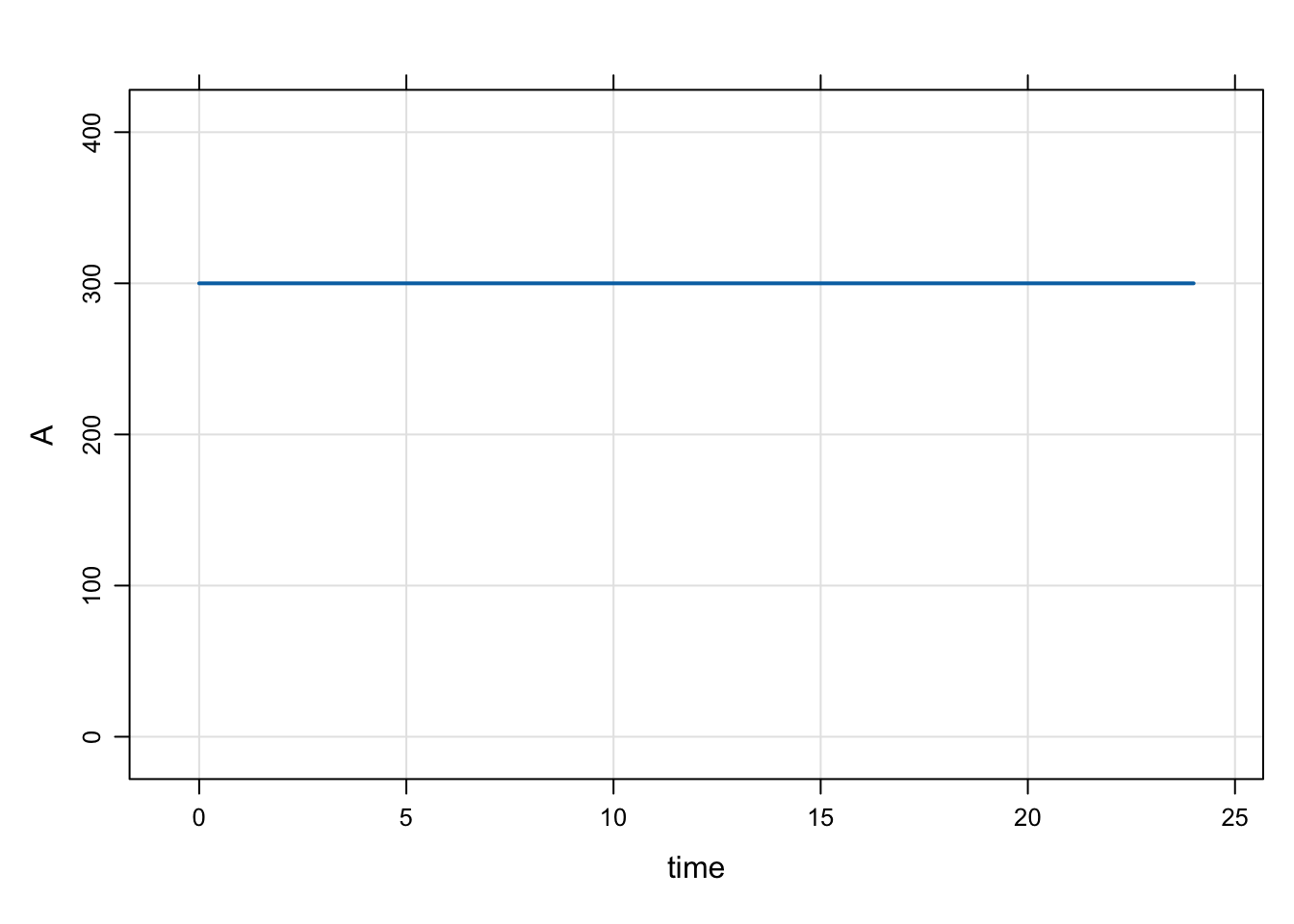## 2.2 Example PK/PD model with initial condition

Just to be clear, there is no need to set any sort of flag to set the initial condition.

``````code <- '
\$PARAM AUC=0, AUC50 = 75, KIN=200, KOUT=5

\$CMT RESP

\$MAIN
RESP_0 = KIN/KOUT;

\$ODE
dxdt_RESP = KIN*(1-AUC/(AUC50+AUC)) - KOUT*RESP;
'``````
``mod <- mcode_cache("init_long2", code)``
``Loading model from cache.``

The initial condition is set to 40 per the values of `KIN` and `KOUT`

``mod %>% mrgsim %>% plot``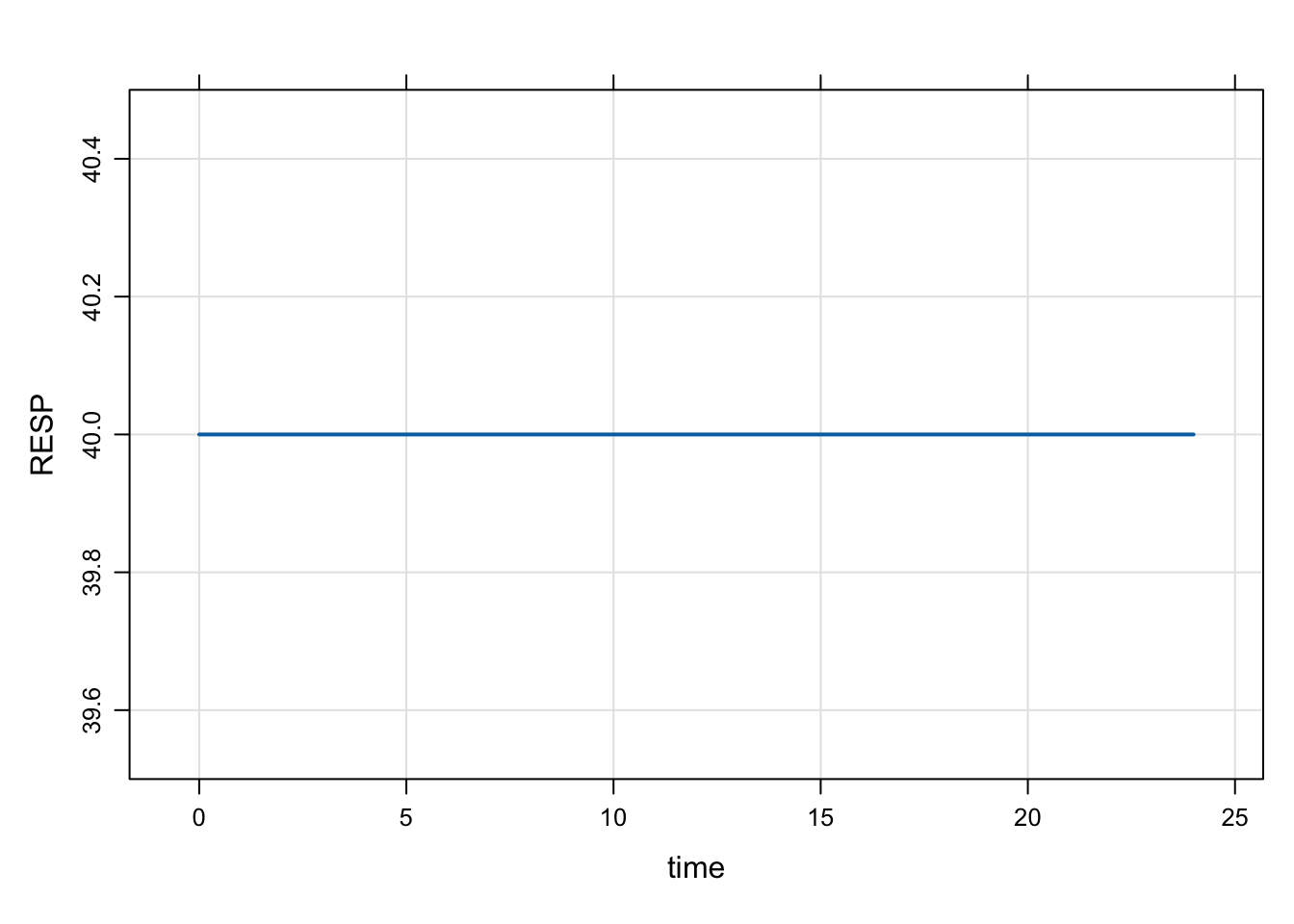Even when we change `RESP_0` in `R`, the calculation in `\$MAIN` gets the final say

``mod %>% init(RESP=1E9) %>% mrgsim``
``````Model:  init_long2
Dim:    25 x 3
Time:   0 to 24
ID:     1
ID time RESP
1:   1    0   40
2:   1    1   40
3:   1    2   40
4:   1    3   40
5:   1    4   40
6:   1    5   40
7:   1    6   40
8:   1    7   40``````

## 2.3 Calling `init` will let you check to see what is going on

• It’s a good idea to get in the habit of doing this when things aren’t clear
• `init` first takes the base initial condition list, then calls `\$MAIN` and does any calculation you have in there; so the result is the calculated initials
``init(mod)``
``````
Model initial conditions (N=1):
name       value . name    value
RESP (1)   40    | . ...   .    ``````
``mod %>% param(KIN=100) %>% init``
``````
Model initial conditions (N=1):
name       value . name    value
RESP (1)   20    | . ...   .    ``````

# 3 Set initial conditions via `idata`

Go back to house model

``````mod <- mrgsolve:::house()
init(mod)``````
``````
Model initial conditions (N=3):
name       value . name       value
CENT (2)   0     | RESP (3)   50
GUT (1)    0     | . ...      .    ``````

Notes

• In `idata` (only), include a column with `CMT_0` (like you’d do in `\$MAIN`).
• When each ID is simulated, the `idata` value will override the base initial list for that subject.
• But note that if `CMT_0` is set in `\$MAIN`, that will override the `idata` update.
``````idata <- expand.idata(CENT_0 = seq(0,25,1))

``````  ID CENT_0
1  1      0
2  2      1
3  3      2
4  4      3
5  5      4
6  6      5``````
``````out <-
mod %>%
idata_set(idata) %>%
mrgsim(end=40)``````
``plot(out, CENT~.)``